# Basen

How many square meters of tiles we need to tile the walls and floor of the pool 15 meters long, six meters wide and two meters?deep

S =  174 m2

### Step-by-step explanation: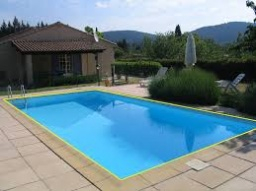Did you find an error or inaccuracy? Feel free to write us. Thank you!Tips to related online calculators
Tip: Our volume units converter will help you with the conversion of volume units.

#### You need to know the following knowledge to solve this word math problem:

We encourage you to watch this tutorial video on this math problem:

## Related math problems and questions:

• Pool tilesThe pool is 25m long, 10m wide, and 160cm deep. How many m2 of tiles will be needed on the walls and the pool? How many tiles are needed when 1 tile has a square shape with a 20cm side? How much does it cost when 1m2 of tiles costs 258 Kc?
• Children's poolChildren's pool at the swimming pool is 10m long, 5m wide and 50cm deep. Calculate: (a) how many m2 of tiles are needed for lining the perimeter walls of the pool? (b) how many hectoliters of water will fit into the pool?
• PoolHow many hl of water is in a cuboid pool (a = 25m, b = 8m) if the area of the wetted walls is 279.2 m2?
• In the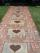In the garden, workers will pave a 1-meter-wide sidewalk with tiles around the block-shaped pool. The dimensions of the bottom of the pool are 8.5 meters and 6 meters. The height of the pool walls is 2 meters. How many m2 of pavement will be laid with til
• PoolThe prism-shaped pool is 2 m deep with a bottom of the isosceles trapezoid with base dimensions of 10 m and 18 m and arm legs 7 m long and 5.7 m long. During the spring cleaning, the bottom and walls of the pool must be painted. How many m2 of paint shoul
• If one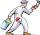If one litre of pant covers an area of 5 m2 how much paint is needed to cover: a) rectangular swimming pool With dimensions 4m x 3m x 2.5m (the Inside walls and the floor only) b) the Inside walls and floor of a cylindrical reservoir with diameter 3m and
• Surface and volume od cuboidContent area of the square base of cuboid is Sp = 36 cm2 and its height 80 mm. Determine its surface area and volume.
• Pool 3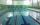How long will fill pool cuboid shape (8m 6m 1.5m) when flows 15 liters/s?
• Pool coating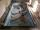How many tiles 25 cm × 15 cm need to coat the bottom and sidewalls of the pool with bottom dimensions 30 m × 5 m, if the pool can fit up to 271500 liters of water?
• TrevorTrevor wishes to tile the floor of his bathroom. The bathroom is rectangular and is 4.2m long and 3.3m wide. The tiles are 30cm by 30cm. Calculate the cost to tile the floor if each tile costs 72 cents.
• Swimming pool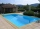A swimming pool 30 meters long is filled with water to a depth of 1 meter at the shallow end, and 5 meters at the deep end and abcd the vertical area of the pool has the shape of a trapezium with the area given by S(abcd)= 1/2 (ab + cd) x ad. What is the
• Water depthHow many square meters of the inside of the pool wet the water if the pool is 25 meters long and 10 meters wide and the water depth is 1.2 meters everywhere.
• Sidewalk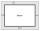In the garden, a 1-meter-wide sidewalk will be paved with tiles around the block-shaped pool. In the picture, the sidewalk is shown in gray. The dimensions of the bottom of the pool are 8.5 meters and 6 meters. The height of the pool walls is 2 meters. Ho
• HectolitersHow deep is the pool if there are 2025 hectoliters of water and the bottom dimensions are a = 15 meters b = 7.5 meters and the water level is up to 9/10 (nine-tenths) of height?
• Rectangle poolFind dimensions of an open pool with a square bottom with a capacity of 32 m3 to have painted/bricked walls with the least amount of material.
• Classic tent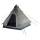The tent has the shape of a triangular prism. The front and rear walls are isosceles triangles with a height of 18 dm and arms 19.5 dm long. The tent is 1.5 m wide and 2 m long. How many square meters of fabric is needed to make a tent? How much air is in
• Swimming pool 5A rectangular inflatable swimming pool is 3 yards long, 2 5/8 yards wide, and  1 3/5 yard tall. What is the volume of the pool? Round to the nearest tenth.